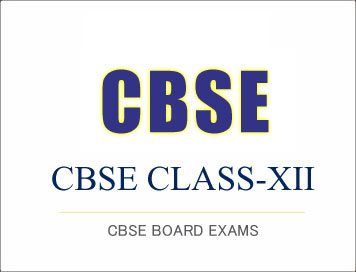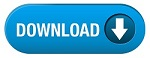# CBSE Class-12 Compartment Exam 2019 : Question Paper- DatabasE Management Applications## CBSE Class-12 Compartment Exam 2019 : Question Paper- DatabasE Management Applications

• Please check that this question paper contains 7 printed pages.
• Code number given on the right hand side of the question paper should be written on the title page of the answer-book by the candidate.
• Please check that this question paper contains 31 questions.
• Please write down the Serial Number of the question before attempting it.
• 15 minute time has been allotted to read this question paper. The question paper will be distributed at 10.15 a.m. From 10.15 a.m. to 10.30 a.m., the students will read the question paper only and will not write any answer on the answer-book during this period.

Time allowed : 2.30 H
hours Maximum Marks : 50

(i) This question paper contains 31 questions, out of which the candidate needs to attempt only 23 questions.
(ii) Question paper is divided into two sections.

SECTION A

• Multiple choice questions / Fill in the blanks/Direct questions : contains total 12 questions of 1 mark each. Answer any 10 questions.
• Very short answer type questions : contains total 7 questions of 2 marks each. Answer any 5 questions.
• Short answer type questions : contains total 7 questions of 3 marks each. Answer any 5 questions.

SECTION B

• Long answer / Essay type questions : contains total 5 questions of 5 marks each. Answer any 3 questions.

## SECTION A

Multiple Choice Questions (Choose the most appropriate option). Attempt any ten questions.
1. USE keyword in MYSQL is used to select a
(i) Table
(ii) Column
(iii) Database
(iv) All of the above

2. A byte code in Java is
(i) Another name for Java source file that contains the information about the hardware.
(ii) A binary code that is generated by JVM for operating system.
(iii) An intermediate code generated by Java compiler for JVM.
(iv) None of these

3. The wild card character used in MYSQL for pattern searching is
(i) * (asterisk)
(ii) % (percentage)
(iii) & (ampersand)
(iv) - (hyphen)

4. Which of the following describes e-commerce ?
(iii) Sale of goods
(iv) All of the above

5. On executing DELETE command, if you get an error ‘‘foreign key constraint’’, what does it imply ?
(i) Foreign key is not defined
(ii) Table is empty
(iii) Connectivity issue
(iv) Data is present in the other table

6. Which type of e-commerce focuses on consumers dealing with each other ?
(i) B2B
(ii) B2C
(iii) C2B
(iv) C2C

7. Which operator performs pattern matching ?
(i) BETWEEN operator
(ii) LIKE operator
(iii) EXISTS operator
(iv) None of the above

8. In SQL which command is used to change a table’s storage characteristics ?
(i) Alter table
(ii) Modify table
(iii) Change table
(iv) All of the above

9. Which data type value is returned by all transcendental math functions ?
(i) int
(ii) float
(iii) double
(iv) long

10. Which of these statements is correct ?
(i) True and false are numeric values 1 and 0.
(ii) True and false are numeric values 0 and 1.
(iii) True is any non-zero value and false is 0.
(iv) True and false are non-numeric values.

11. Which of these packages is used for text formatting in Java programming language ?
(i) Java.text
(ii) Java.awt
(iii) Java.awt.text
(iv) Java.io

12. ___________ is the use of electronic i.e. information and communication technique tools for governance by the government for the citizens.
(i) e-learning
(ii) e-governance
(iii) e-payment
(iv) e-commerce